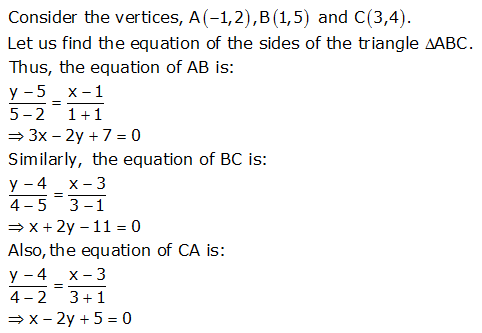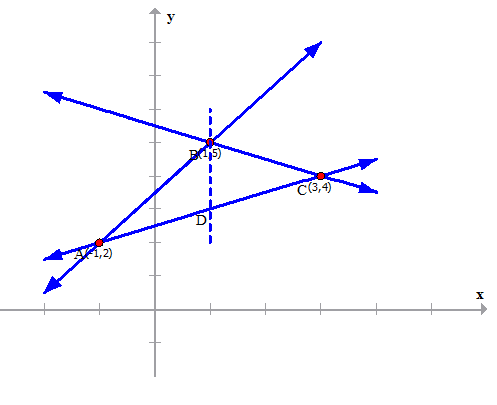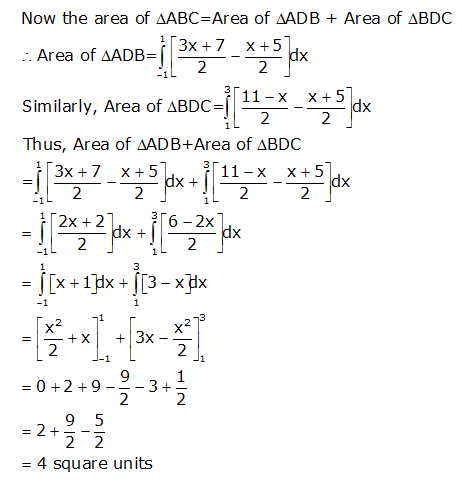Request a call back

<p>Using intergration find the area bounded by the triangle whose vertices are given by A (1,0), (2,2) and (3,1).</p> <p>&nbsp;</p> <p>My doubt id that how do we get the equation in terms of 'y' so that we are easy to intergrate using: intergral of y dx&nbsp;</p>
Asked by Shanmuga Priya | 19 Mar, 2014, 12:13: PMExpert Answer
First find the equation of the lines AB, BC and CA using two points formula.

Then write y in terms of x for all the equations.

Draw a rough graph for the triangle.

Mark the intersection points.

Find the difference of ordinates, that is, find the difference of the two equations of the lines which are intersecting.

Then integrate the function.

We are providing  a solution to a different question. You can refer to this question and follow the same steps.Answered by | 03 Apr, 2014, 12:14: PM

## Concept Videos

CBSE 12-science - Maths
Asked by prasannavvss5 | 27 Sep, 2020, 01:00: PMANSWERED BY EXPERT
CBSE 12-science - MathsAsked by NITISH DAS | 15 Apr, 2020, 12:13: AMANSWERED BY EXPERT
CBSE 12-science - Maths
Pl ansANSWERED BY EXPERT
CBSE 12-science - Maths
Plz answer 23rd sum with easy  graphAsked by lovemaan5500 | 29 Nov, 2019, 04:18: PMANSWERED BY EXPERT
CBSE 12-science - Maths
What will be the answer for this question?Asked by dineshchem108 | 05 Jul, 2019, 05:58: PMANSWERED BY EXPERT
CBSE 12-science - MathsAsked by dineshchem108 | 05 Jul, 2019, 05:56: PMANSWERED BY EXPERT
CBSE 12-science - Maths
Asked by bjayanta | 20 Nov, 2018, 02:05: PMANSWERED BY EXPERT
CBSE 12-science - MathsANSWERED BY EXPERTANSWERED BY EXPERTANSWERED BY EXPERT